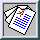Advances in DNS/LES, edited by C. Liu and Z. Liu, pp. 57-68 (Greydon Press, Columbus, 1997)

### Decaying turbulence in two and three dimensions

J. R. Chasnov
The Hong Kong University of Science and Technology, Hong Kong

A review of decaying isotropic turbulence in two and three dimensions is presented. In both dimensions, exact results can be obtained by assuming that for small Reynolds numbers the nonlinear convective terms in the equation of motion are negligible. These analytical results obtained from a linear equation demonstrate explicitly that the decay laws depend on the form of the energy spectrum near zero wavenumber. In three dimensions, high Reynolds number decay laws can be obtained by assuming that these laws continue to depend on invariant or near-invariant low wavenumber spectral coefficients but become independent of viscosity. Results of numerical simulations are presented which smoothly connect the asymptotically high and low Reynolds number solutions. In two dimensions however, high Reynolds number solutions can not be as easily obtained since the dominant nonlinear energy cascade is from small-to-large scales so that an assumption such as the invariance or near-invariance of low wavenumber spectral coefficients becomes untenable. However, exact analytical results in two dimensions can be determined at a transitional Reynolds number, below which final period of decay solutions result and above which the turbulence evolves with increasing Reynolds number.

Full Text: [PDF (514 KB) GZipped PS(146 KB)]